## Selina Concise Mathematics Class 6 ICSE Solutions Chapter 31 Recognition of Solids

Selina Publishers Concise Mathematics Class 6 ICSE Solutions Chapter 31 Recognition of Solids

APlusTopper.com provides step by step solutions for Selina Concise ICSE Solutions for Class 6 Mathematics. You can download the Selina Concise Mathematics ICSE Solutions for Class 6 with Free PDF download option. Selina Publishers Concise Mathematics for Class 6 ICSE Solutions all questions are solved and explained by expert mathematic teachers as per ICSE board guidelines.

### Recognition of Solids Exercise 31 – Selina Concise Mathematics Class 6 ICSE Solutions

Question 1.
Identify the nets which can be used to form cubes :Solution:
Nets for a cube are (ii), (iii) and (iv).

Question 2.
Draw at least three different nets for making cube.
Solution: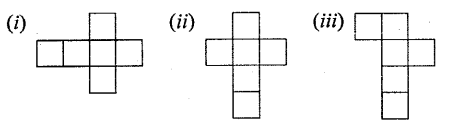Question 3.
The dimensions of a cuboid are 6 cm, 4 cm and 3 cm. Draw two different types of oblique sketches for this cuboid.
Solution: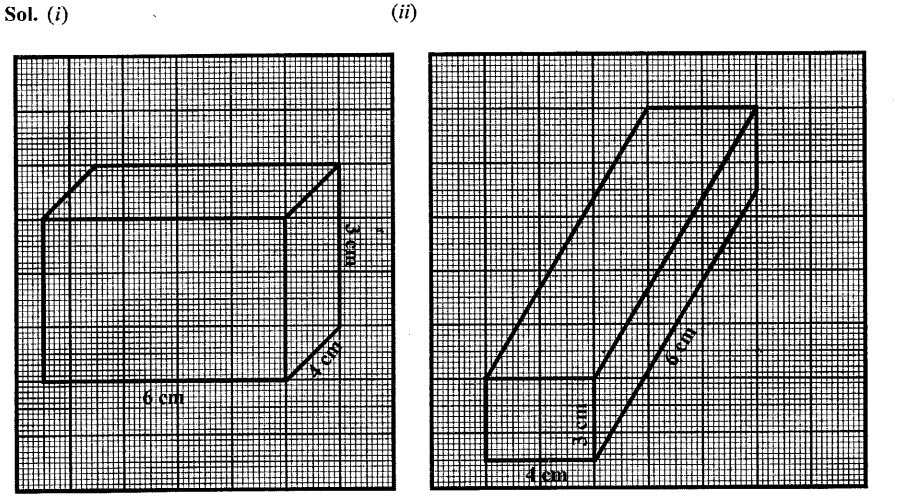Question 4.
Two cubes, each with 3 cm edge, are placed side by side to form a cuboid. For this cuboid, draw :
(i) an oblique sketch
(ii) an isometric sketch.

Solution:Question 5.
The figure, given below, shows shadows of some 3D objects, when seen under the lamp of an overhead projector :In each case, name the object.
Solution:Question 6.
Look at the solids, drawn below, and fill the given chart.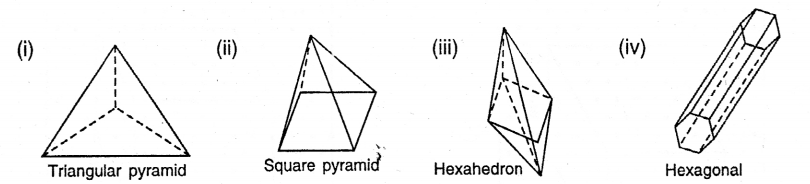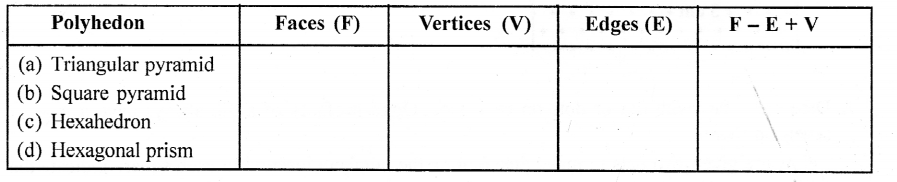Solution: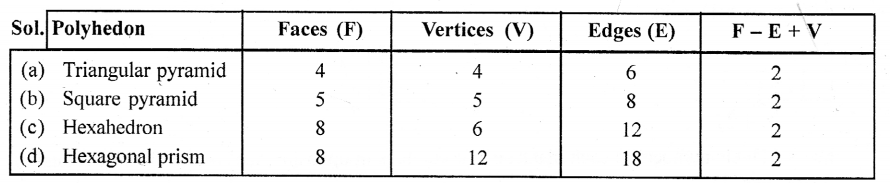P. Q. Using Euler’s formula, find the values of a, b, c and d.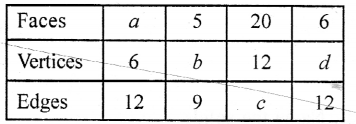Solution: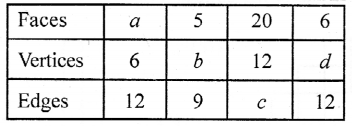(i) a + 6 — 12 = 2 ⇒a = 2- 6+12 = 14-6 = 8
(ii) b + 5- 9 = 2 ⇒b-2 + 9-5 = 6
(iii) 20 + 12 – c = 2 ⇒ 32 – c = 2 ⇒ c = 32 – 2 ⇒ c = 30
(iv) 6 + d – 12 = 2⇒ d-6 = 2 ⇒ d = 2 + 6 = 8

P.Q. Using an isometric dot paper, draw :
(i) a cube with each edge 3 cm.
(ii) a cuboid measuring 5 cm x 4 cm x 3 cm.

Solution: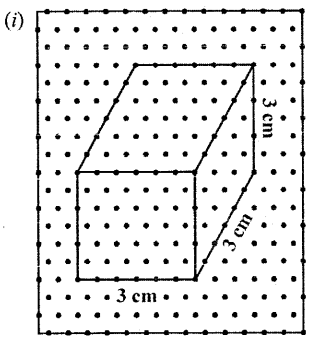Question 7.
Dice are cubes with dot or dots on each face. Opposite faces of a die always have a total of seven on them.
Below are given two nets to make dice (cube), the numbers inserted in each square indicate the number of dots in it.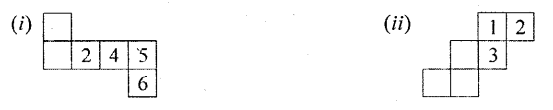Insert suitable numbers in each blank so that numbers in opposite faces of the die have a total of seven dots.
Solution: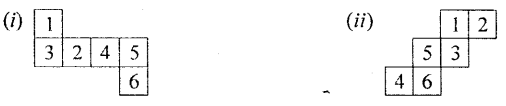Question 8.
The following figure represents mets of some solids. Name the solids.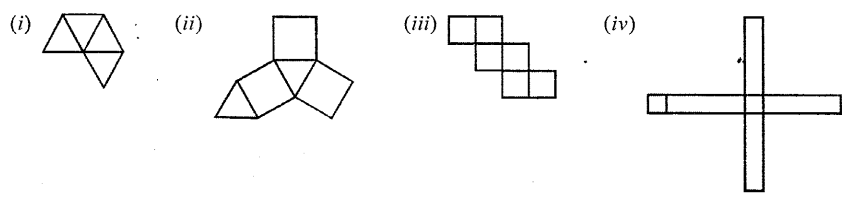Solution:
The given nets are of the solid as given below :
(i) Tetrahedron
(ii) Triangular prism
(iii) Cube
(iv) Cuboid